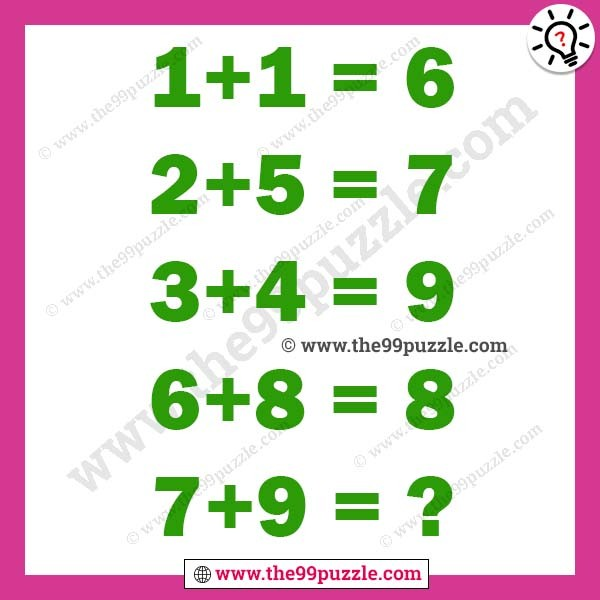# Maths logical equation brain teaser with answer – Puzz168

Maths logical equation brain teaser with answer. Most of the people fail to answer this simple math logic equation. If you are genius people you can easily solve the last equation.

1+1=6

2+5=7

3+4=9

6+8=8

7+9=?###### Explanation:

1+1=ONE+ONE=3+3=6

2+5=TWO+FIVE=3+4=7

3+4=THREE+FOUR=5+4=9

6+8=SIX+EIGHT=3+5=8

7+9=SEVEN+NINE=5+4=9

1.